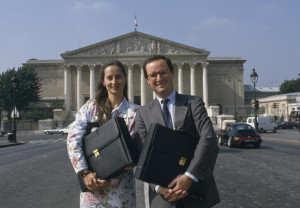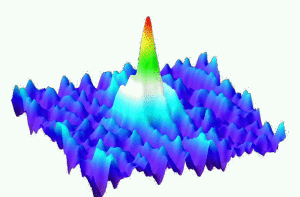# Month: April 2012En 1965 déjà, Pierre Dac présentait sa candidature aux présidentielles, au nom du Mouvement Ondulatoire Unifié (MOU) dont la devise était : « Les temps sont durs, vive le MOU ! »Last Updated on 2014-06-17In quantum mechanics, the spin-statistics theorem states that sub-atomic particles are either bosons or fermions. Photons are bosons, while electrons are fermions. Bosons have integer spin, and the wave function of a system of two bosons is symmetric. Fermions have half integer spin, and the wave function of a system of two fermions is antisymmetric. Bosons can occupy the same quantum state, and obey to the Bose-Einstein statistics. Fermions cannot occupy the same quantum state (Pauli exclusion principle), and obey to the Fermi-Dirac statistics. These statistics can be retained using some elementary combinatorics, in the spirit of the famous Maxwell-Boltzmann statistics.

Maxwell-Boltzmann statistics. We have ${m}$ energy levels ${E_1,\ldots,E_m}$ and ${n}$ distinguishable particles. If ${n_i}$ is the number of ${E_i}$ particles then ${n=n_1+\cdots+n_m}$ and the average energy of configuration ${(n_1,\ldots,n_m)}$ is ${E=n_1E_1+\cdots+n_mE_m}$. There are

$\binom{n}{n_1,\ldots,n_m}=n!\prod_{i=1}^m\frac{1}{n_i!}$

ways to obtain the configuration ${(n_1,\ldots,n_m)}$ (multinomial coefficient: throw ${n}$ distinguishable balls into ${m}$ boxes). In order to get an additive measurement of the degree of freedom, we may take the logarithm. The additive degree of freedom per particle is then ${\frac{1}{n}\log\binom{n}{n_1,\ldots,n_m}}$. Now, if ${n\rightarrow\infty}$ with ${n_i/n\rightarrow p_i}$ for every ${1\leq i\leq n}$ then ${p_1+\cdots+p_m=1}$, and thanks to the Stirling formula, the asymptotic degree of freedom per particle is given by

$-\sum_{i=1}^mp_i\log(p_i).$

This quantity is known as the Boltzmann entropy. Maximizing this entropy subject to the fixed average energy constraint ${E=\sum_{i=1}^mp_iE_i}$ gives

$p_i=Z^{-1}e^{-\beta E_i}$

where the Lagrange multiplier ${\beta>0}$ can be interpreted to be proportional to an inverse temperature, and were ${Z=\sum_{i=1}^me^{-\beta E_i}}$ is a normalizing factor.

As pointed out by Gibbs, the reasoning above is faulty. Namely, if we consider a system formed by ${n+n’}$ particles, then the logarithmic degree of freedom is actually not additive, violating the second principle of thermodynamics. A way to solve this paradox is to assume that the particles are undistinguishable. This makes the combinatorics trivial since for every configuration ${(n_1,\ldots,n_m)}$ there is a unique way to throw our undistinguishable particles into the ${m}$ boxes with ${n_i}$ particles in each box for every ${1\leq i\leq m}$. To escape from this triviality, we assume that each energy level ${E_i}$ has ${s_i}$ states, in other words that box ${i}$ has ${s_i}$ sub-boxes. The number of ways to obtain configuration ${(n_1,\ldots,n_m)}$ is then

$\prod_{i=1}^m\frac{(n_i+s_i-1)!}{n_i!(s_i-1)!}.$

If ${s_1=\cdots=s_m=1}$ then this number is ${1}$. If ${n_i\ll s_i}$ then thanks to the Stirling formula, the number of ways to obtain configuration ${(n_1,\ldots,n_m)}$ is

$\approx\prod_{i=1}^m\frac{s_i^{n_i}}{n_i!}.$

The variational reasoning used for the distinguishable case leads then to another distribution for the energy levels, known as the Maxwell-Boltzmann statistics:

$p_i\approx Z^{-1}s_ie^{-\beta E_i}.$

When ${s_1=\cdots=s_m}$ we recover the same distribution.

Bose-Einstein statistics. We follow the reasoning used for the Maxwell-Boltzmann statistics, without the assumption ${n_i\ll s_i}$. This leads to

$p_i\approx Z^{-1}\frac{s_i}{e^{\beta E_i-\alpha}-1}.$

Fermi-Dirac statistics. Suppose this time that at most one particle can occupy a state (i.e. a sub-box). The number of ways to obtain configuration ${(n_1,\ldots,n_m)}$ is this time

$\prod_{i=1}^m\frac{s_i!}{n_i!(s_i-n_i)!},$

$p_i\approx Z^{-1}\frac{s_i}{e^{\beta E_i-\alpha}+1}.$The success of computers is largely due to the existence of efficient algorithms for solving numerical and combinatorial problems. These algorithms are used billion of times per second. Many users (even among scientists) do not know them. One may find a list of algorithms on Wikipedia. Depending on your tastes, you may distinguish some favorite ones, for their simplicity, elegance, or versatility. Here are some few of my favorite algorithms and paradigms:

Ironically, the most used algorithms (if we measure say in Megawatt) concern coding, compression, cryptography, linked with what is often identified as “pure mathematics” (finite fields algebra, discrete linear algebra, discrete harmonic analysis). This does not mean that numerical algorithms, identified as “applied mathematics”, are not used! To me, what matters is the depth and beauty of concepts and the efficiency and versatility of implementations.

• Iterative and non-iterative
• Exact and approximate
• Stochastic and deterministic
• Integer and numerical
• Euclid and Archimedes

Last Updated on 2012-04-29

Syntax · Style · .